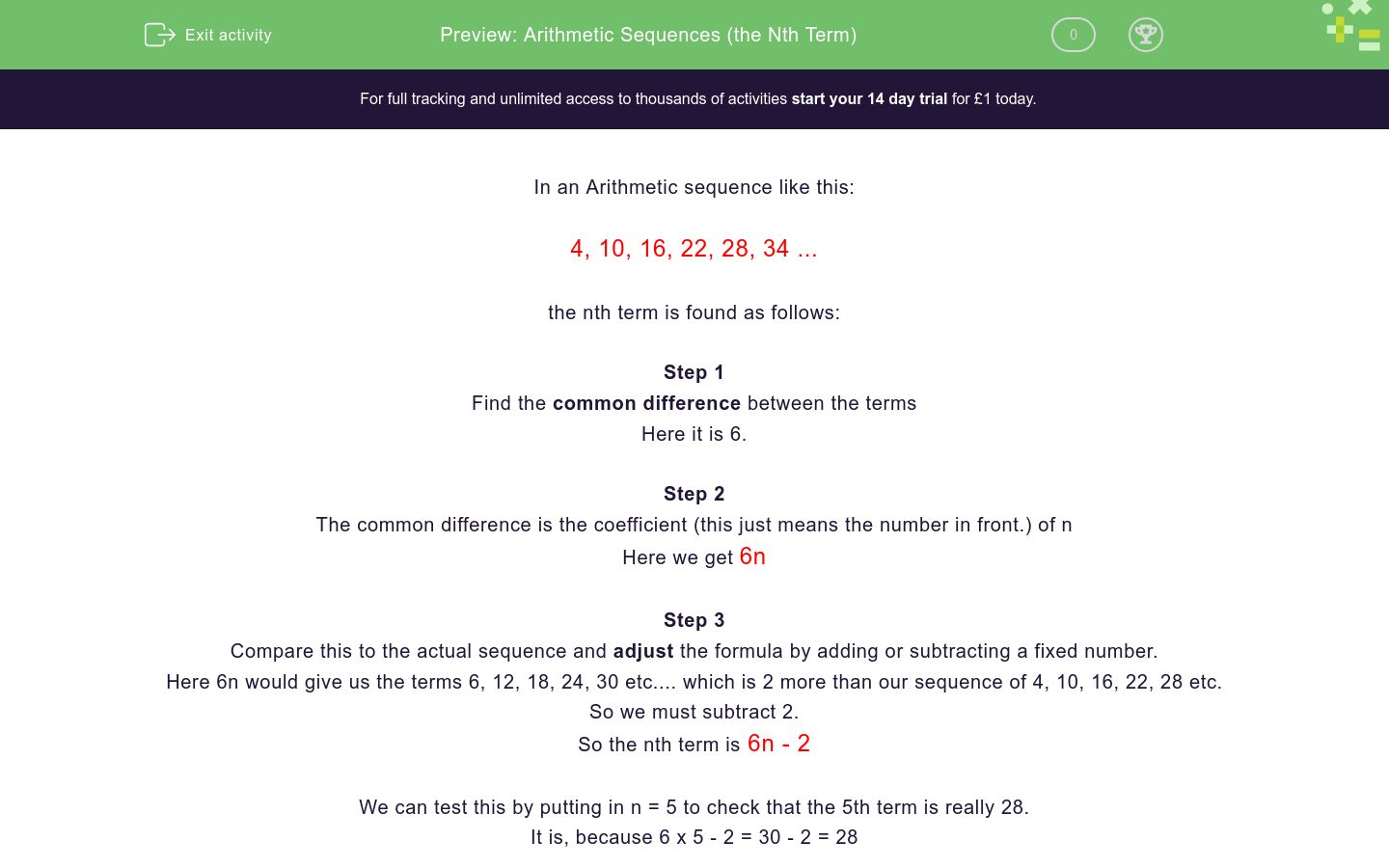# Arithmetic Sequences (the Nth Term)

In this worksheet, students find the Nth term in simple linear sequences.Key stage:  KS 3

Curriculum topic:   Algebra

Curriculum subtopic:   Use Sequences to Find the nth Term

Difficulty level:### QUESTION 1 of 10

In an Arithmetic sequence like this:

4, 10, 16, 22, 28, 34 ...

the nth term is found as follows:

Step 1

Find the common difference between the terms

Here it is 6.

Step 2

The common difference is the coefficient (this just means the number in front.) of n

Here we get 6n

Step 3

Compare this to the actual sequence and adjust the formula by adding or subtracting a fixed number.

Here 6n would give us the terms 6, 12, 18, 24, 30 etc.... which is 2 more than our sequence of 4, 10, 16, 22, 28 etc.

So we must subtract 2.

So the nth term is 6n - 2

We can test this by putting in n = 5 to check that the 5th term is really 28.

It is, because 6 x 5 - 2 = 30 - 2 = 28

For this worksheet, It is important that you do not put spaces in your answer (For example, you would need to put in 5n-3 instead of 5n  -  3. If you put in spaces, the system will mark it as wrong.

Work out the nth term in this sequence:

1, 2, 3, 4, 5, 6 ...

Work out the nth term in this sequence:

2, 4, 6, 8, 10, 12 ...

Work out the nth term in this sequence:

5, 10, 15, 20, 25, 30 ...

Work out the nth term in this sequence:

2, 3, 4, 5, 6, 7 ...

Work out the nth term in this sequence:

1, 3, 5, 7, 9, 11  ...

Work out the nth term in this sequence:

1, 4, 7, 10, 13, 16 ...

Work out the nth term in this sequence:

4, 7, 10, 13, 16, 19 ...

Work out the nth term in this sequence:

1, 6, 11, 16, 21, 26 ...

Work out the nth term in this sequence:

10, 17, 24, 31, 38, 45 ...

Work out the nth term in this sequence:

9, 13, 17, 21, 25, 29 ...

• Question 1

Work out the nth term in this sequence:

1, 2, 3, 4, 5, 6 ...

n
EDDIE SAYS
Common difference is 1.
• Question 2

Work out the nth term in this sequence:

2, 4, 6, 8, 10, 12 ...

2n
EDDIE SAYS
Common difference is 2.
• Question 3

Work out the nth term in this sequence:

5, 10, 15, 20, 25, 30 ...

5n
EDDIE SAYS
Common difference is 5.
• Question 4

Work out the nth term in this sequence:

2, 3, 4, 5, 6, 7 ...

n+1
EDDIE SAYS
Common difference is 1.
• Question 5

Work out the nth term in this sequence:

1, 3, 5, 7, 9, 11  ...

2n-1
EDDIE SAYS
Common difference is 2.
• Question 6

Work out the nth term in this sequence:

1, 4, 7, 10, 13, 16 ...

3n-2
EDDIE SAYS
Common difference is 3.
• Question 7

Work out the nth term in this sequence:

4, 7, 10, 13, 16, 19 ...

3n+1
EDDIE SAYS
Common difference is 3.
• Question 8

Work out the nth term in this sequence:

1, 6, 11, 16, 21, 26 ...

5n-4
EDDIE SAYS
Common difference is 5.
• Question 9

Work out the nth term in this sequence:

10, 17, 24, 31, 38, 45 ...

7n+3
EDDIE SAYS
Common difference is 7.
• Question 10

Work out the nth term in this sequence:

9, 13, 17, 21, 25, 29 ...

4n+5
EDDIE SAYS
Common difference is 4.
---- OR ----

Sign up for a £1 trial so you can track and measure your child's progress on this activity.

### What is EdPlace?

We're your National Curriculum aligned online education content provider helping each child succeed in English, maths and science from year 1 to GCSE. With an EdPlace account you’ll be able to track and measure progress, helping each child achieve their best. We build confidence and attainment by personalising each child’s learning at a level that suits them.

Get started